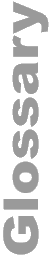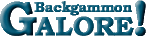Janowski's FormulaJanowski's Formula A formula devised by Rick Janowski for estimating match equity (1) at a given score. If d is the difference in match score and t is the number of points (4) the trailing player has to go, then the probability of the leading player winning the match is .5 + .85d / (t+6).  See also: Neil's Numbers and Turner's Formula.

 Help   •   About the Glossary   •   List of Terms   •   Full Glossary